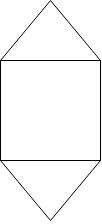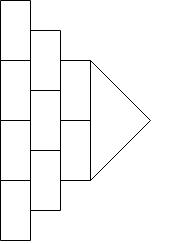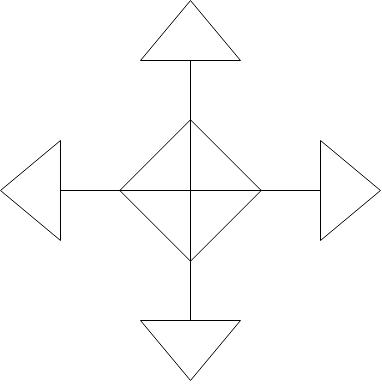# Line symmetry

### Line symmetry

Objects which have line symmetry or reflection symmetry can form symmetrical images where they are cut by an imaginary line that is called line of symmetry. An object can have more than one lines of symmetry.

#### Lessons

• Introduction
a)
1. What is line symmetry?
2. What is rotational symmetry?

• 1.
Find and draw all the lines of symmetry for each figure.
a)b)c)